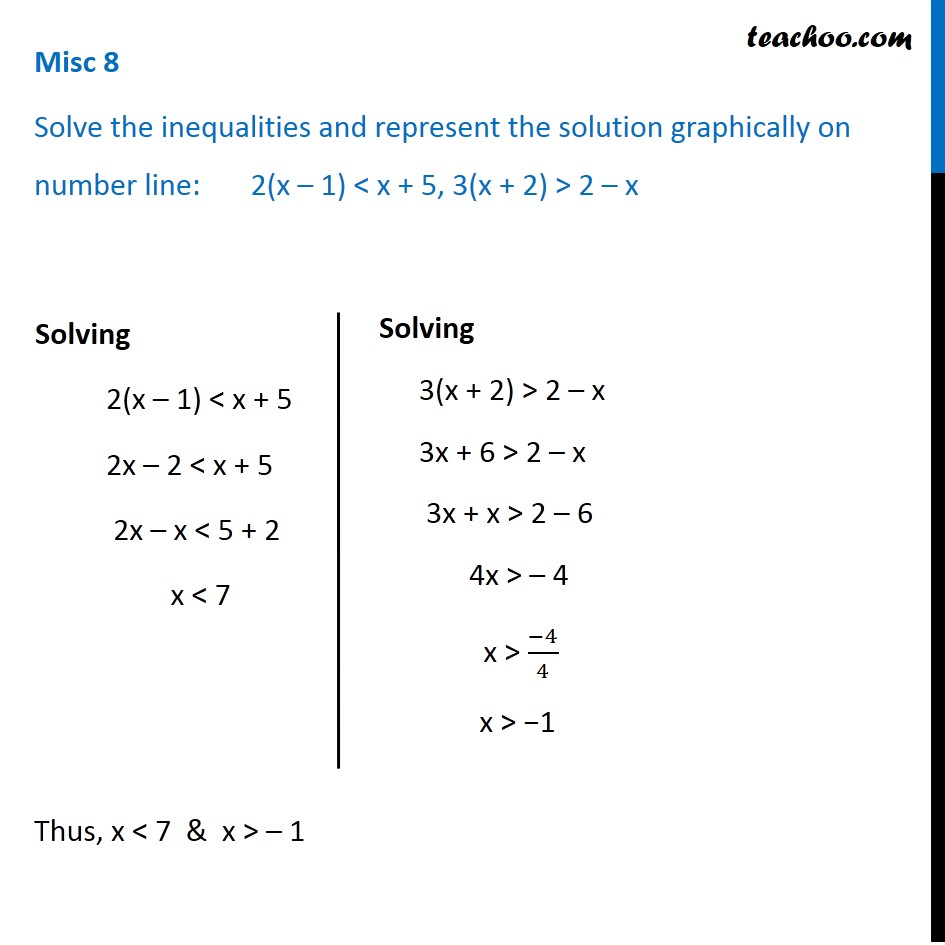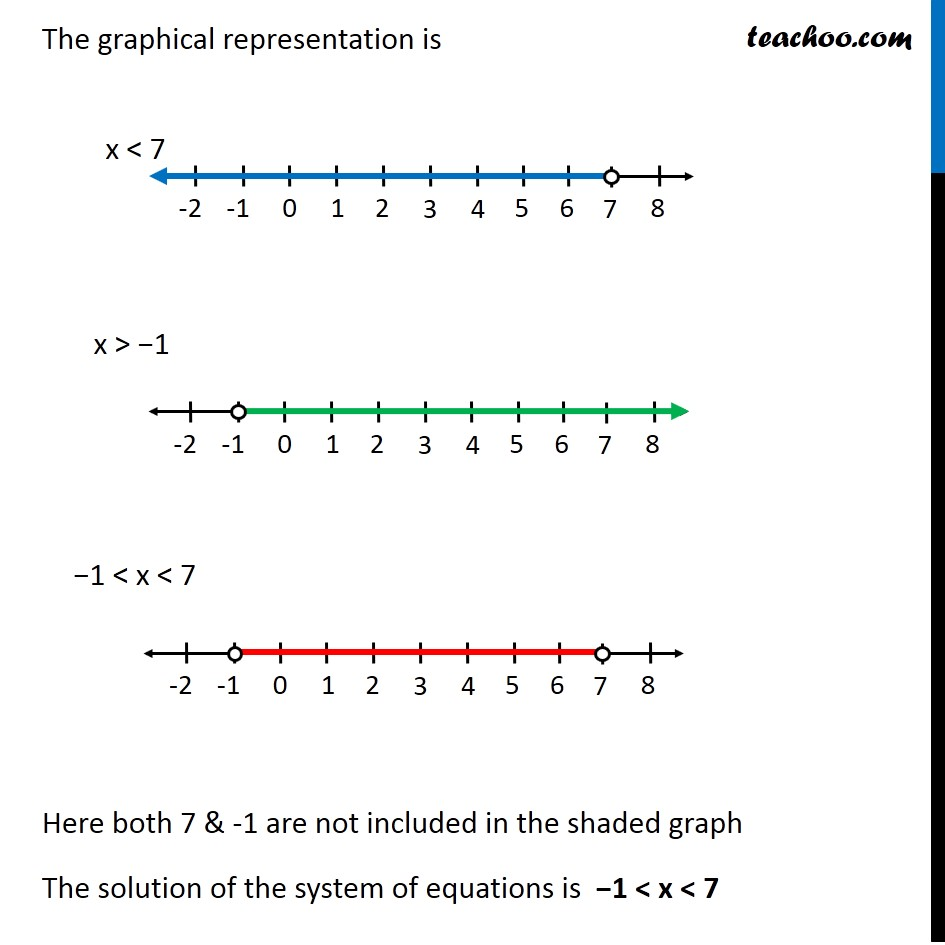Miscellaneous

Chapter 6 Class 11 Linear Inequalities
Serial order wiseGet live Maths 1-on-1 Classs - Class 6 to 12

### Transcript

Misc 8 Solve the inequalities and represent the solution graphically on number line: 2(x – 1) < x + 5, 3(x + 2) > 2 – x Solving 2(x – 1) < x + 5 2x – 2 < x + 5 2x – x < 5 + 2 x < 7 Solving 3(x + 2) > 2 – x 3x + 6 > 2 – x 3x + x > 2 – 6 4x > – 4 x > (−4)/4 x > −1 Thus, x < 7 & x > – 1 The graphical representation is Here both 7 & -1 are not included in the shaded graph The solution of the system of equations is −1 < x < 7